# Area of a shape

 1 Introduction to Area 2 How to Calculate the Area 3 Area of a Square 4 Area of a Rectangle 5 Area of a Circle 6 Area of Shapes - Formula 7 Solved Examples for Calculating Area 8 Area Measuring Units 9 Challenging Questions on Area of Shapes 10 Practice Questions on Area of Shapes 11 Tips and Tricks 12 Maths Olympiad Sample Papers 13 Important Topics of Area of a Shape 14 Frequently Asked Questions (FAQs)

We at Cuemath believe that Math is a life skill. Our Math Experts focus on the “Why” behind the “What.” Students can explore from a huge range of interactive worksheets, visuals, simulations, practice tests, and more to understand a concept in depth.

Book a FREE trial class today! and experience Cuemath's LIVE Online Class with your child.

## Introduction to Area

The word "area" means vacant surface in Latin.

Area can be defined as the amount of space covered by a flat surface of a particular shape.

It is measured in terms of the "number of" square units (square centimeters, square inches, square feet, etc.)

Most objects or shapes have edges and corners.

The length and breadth of these edges are considered while calculating the area of a specific shape.

## How to Calculate the Area

We use a grid to calculate the area of a shape.

The grid is made up of many squares of sides 1 unit by 1 unit.

The area of each of these squares is 1 square unit.

Hence, each square is known as a unit square.

Look at the figure shown below.

Let us find the area of the shape drawn in the grid.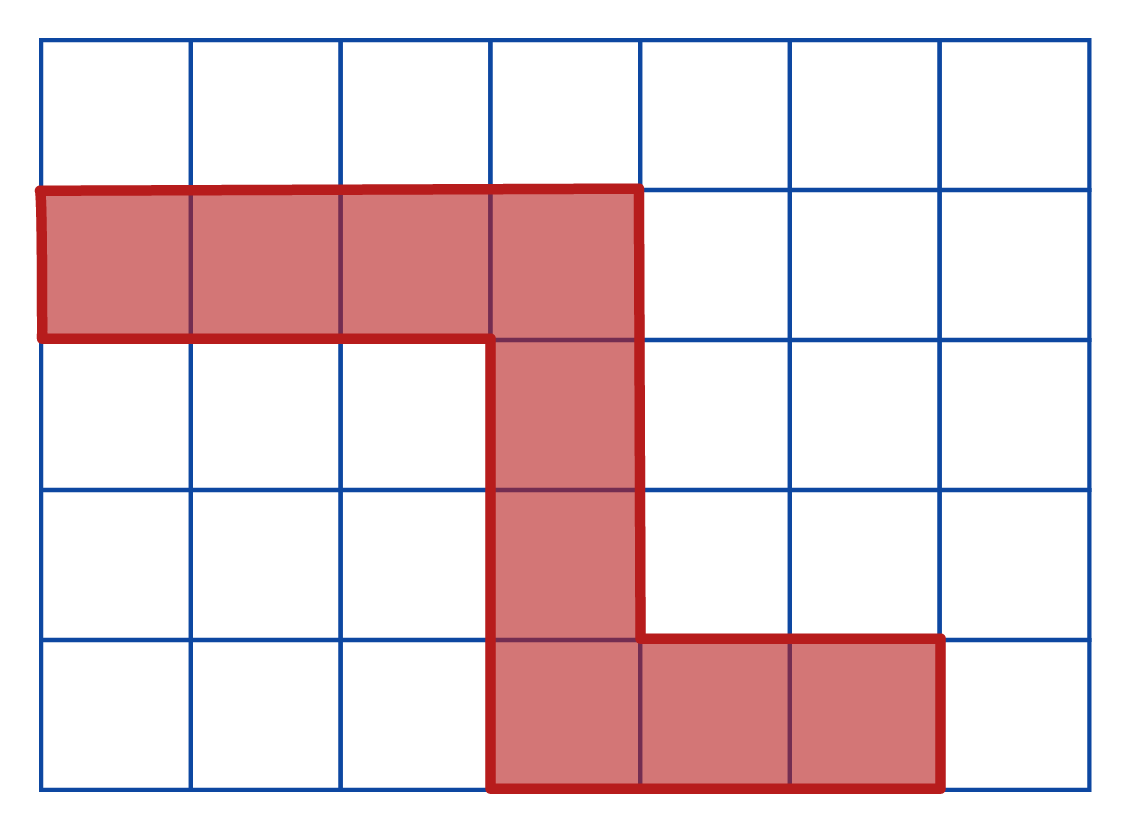The area of this shape is the number of shaded unit squares.

Let's assume that each side of the unit square is 1 unit.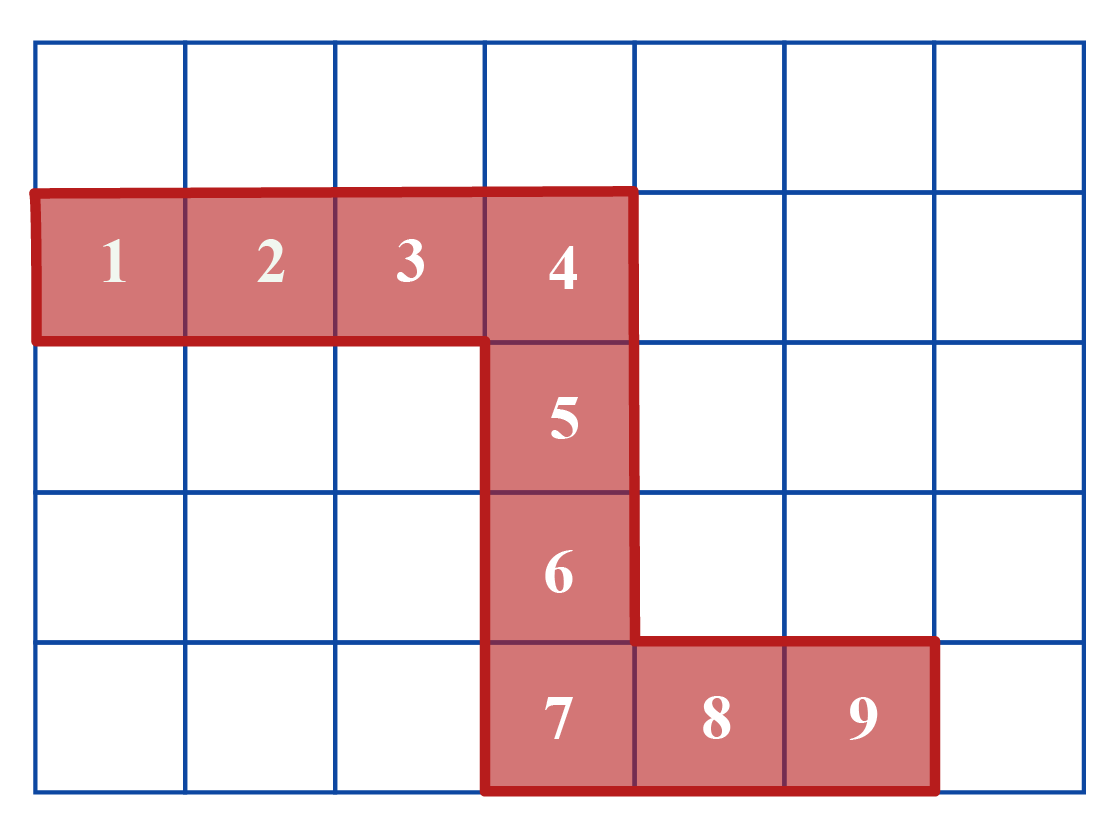Thus, Area = 9 sq. units.

When the shape does not occupy the complete unit square, we can approximate and find its value.

If it occupies about $$\dfrac{1}{2}$$ of the unit square, we can combine two such halves to form an area of 1 sq. unit.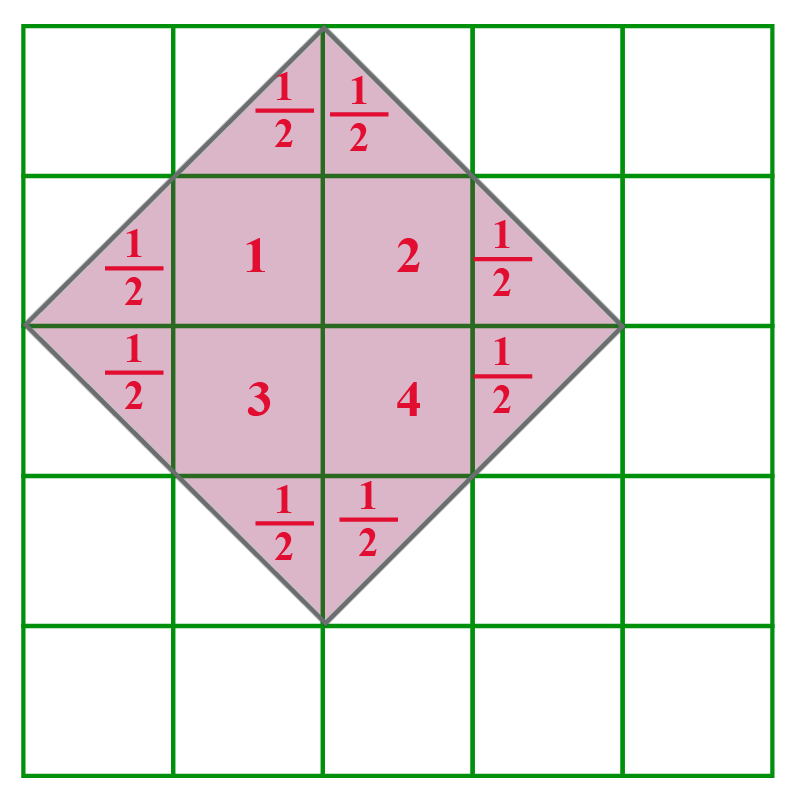Therefore, the area occupied by the shape = 4 full squares and 8 half squares

Together this forms an area of 8 sq. units.

If the shaded region is less than $$\dfrac{1}{2}$$, we can omit those parts.

For regular shapes, we have certain formulas to calculate their area.

Note that this is only an approximate value.

The simulation below generates some shapes.

Can you count the squares and find its area?

## Area of a Square

The area of a square is the space occupied by a square.

Look at the orange square shown in the grid below.

It occupies 25 square units.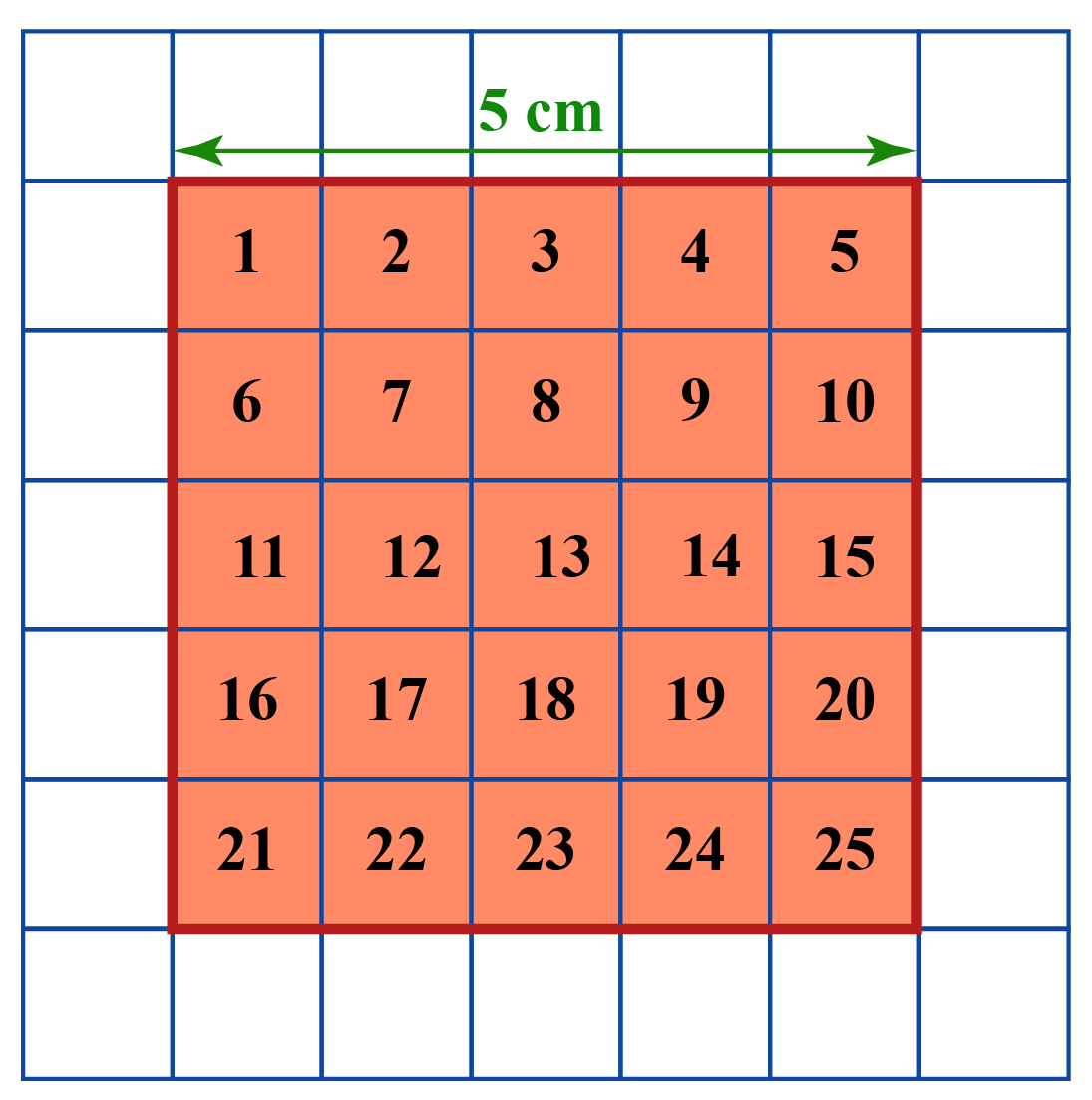From the figure, we can observe that the length of each side of the orange square is 5 units.

Therefore, the area of the square is the product of its sides.

Area of square = side $$\times$$ side = 25 square units

 \begin{align} \text {Area of a Square} = s \!\times\! s \text{ units}^2 \end{align}

## Area of a Rectangle

The area of a rectangle is the space occupied by a rectangle.

Consider the yellow rectangle in the grid.

It has occupied 6 units.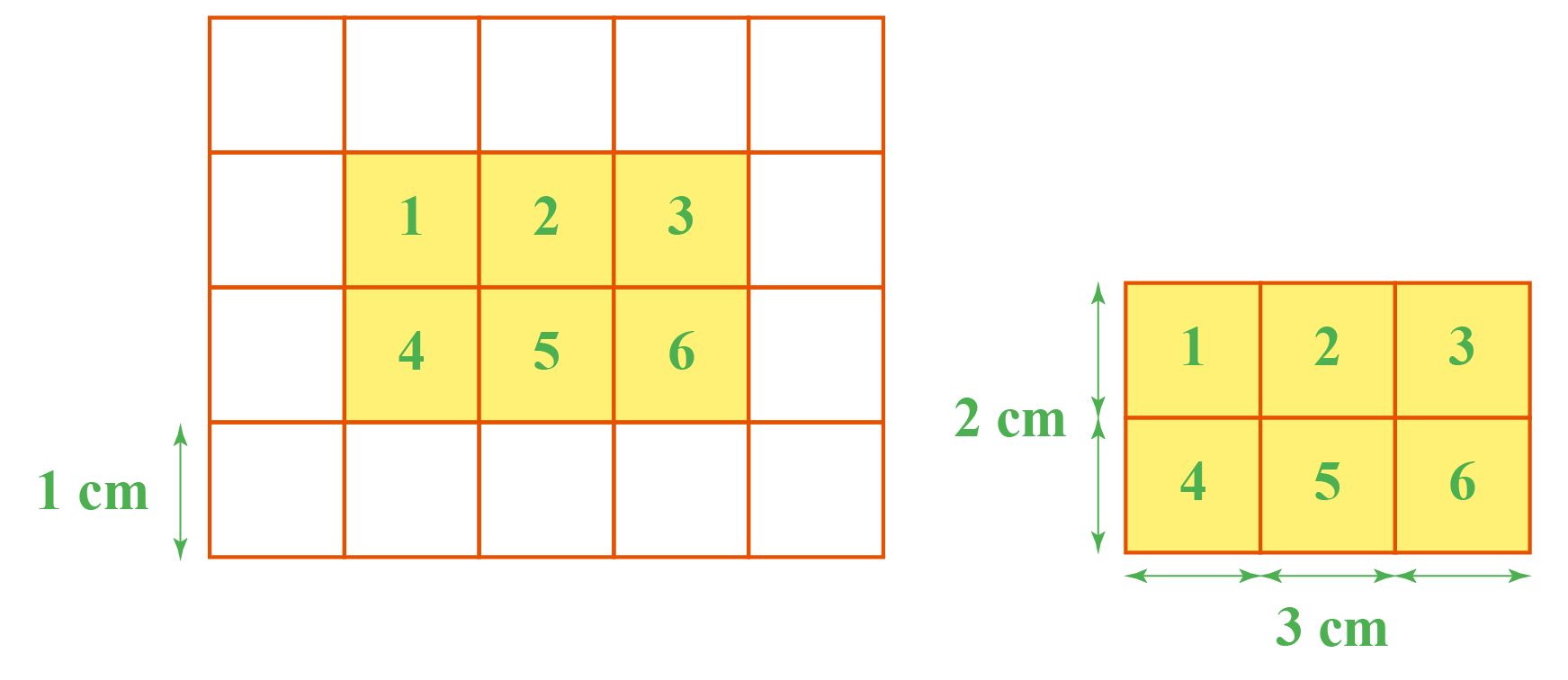In the above example, the area of the rectangle whose length is 2 units and breadth is 3 units is $$6\text{ unit}^2$$.

We have, $$2 \times 3 = 6$$

The area of a rectangle is obtained by multiplying its length and breadth which is the same as counting the unit squares.

Thus, the formula for the area, $$A$$, of a rectangle whose length and breadth are $$l$$ and $$b$$ respectively is the product $${l \times b}$$.

 \begin{align}\text{Area of a Rectangle} = l \!\times\! b \text{ units}^2 \end{align}

## Area of a Circle

A circle is a curved shape.

The area of a circle is the amount of space enclosed within the boundary of a circle.

Learn more about $$\pi$$ and radius before we get to the formula for the area of a circle.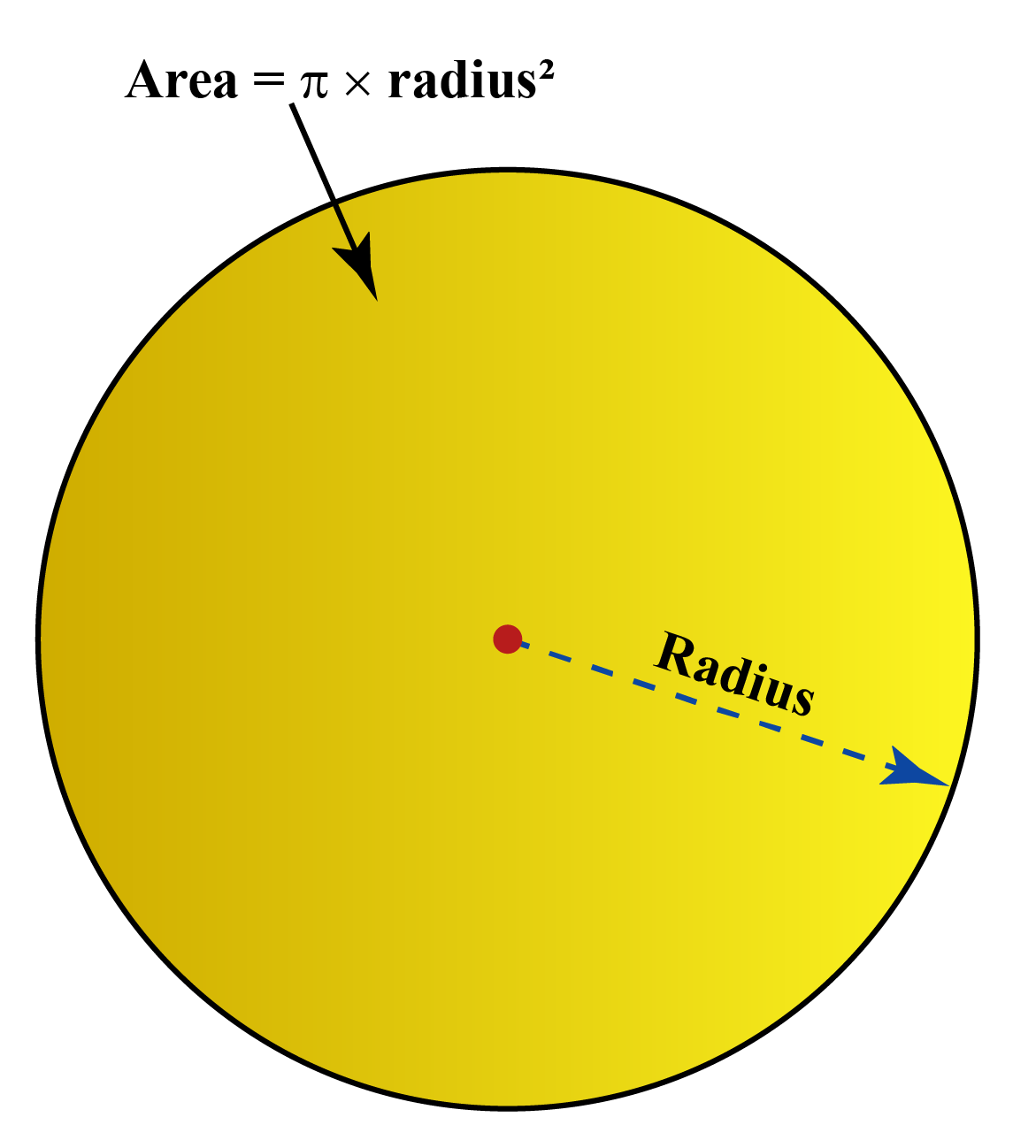The area of circle is given by the formula:

 $$\pi \text{r}^2$$

where $$r$$ is the radius of the circle and $$\text{Pi} - \pi$$  is the mathematical constant whose value is approximated to $$3.14$$  or $$\frac{22}{7}$$

## Area of Shapes - Formula

Here is the list of formulas for the area of shapes.

Shape Area of Shapes - Formula
Square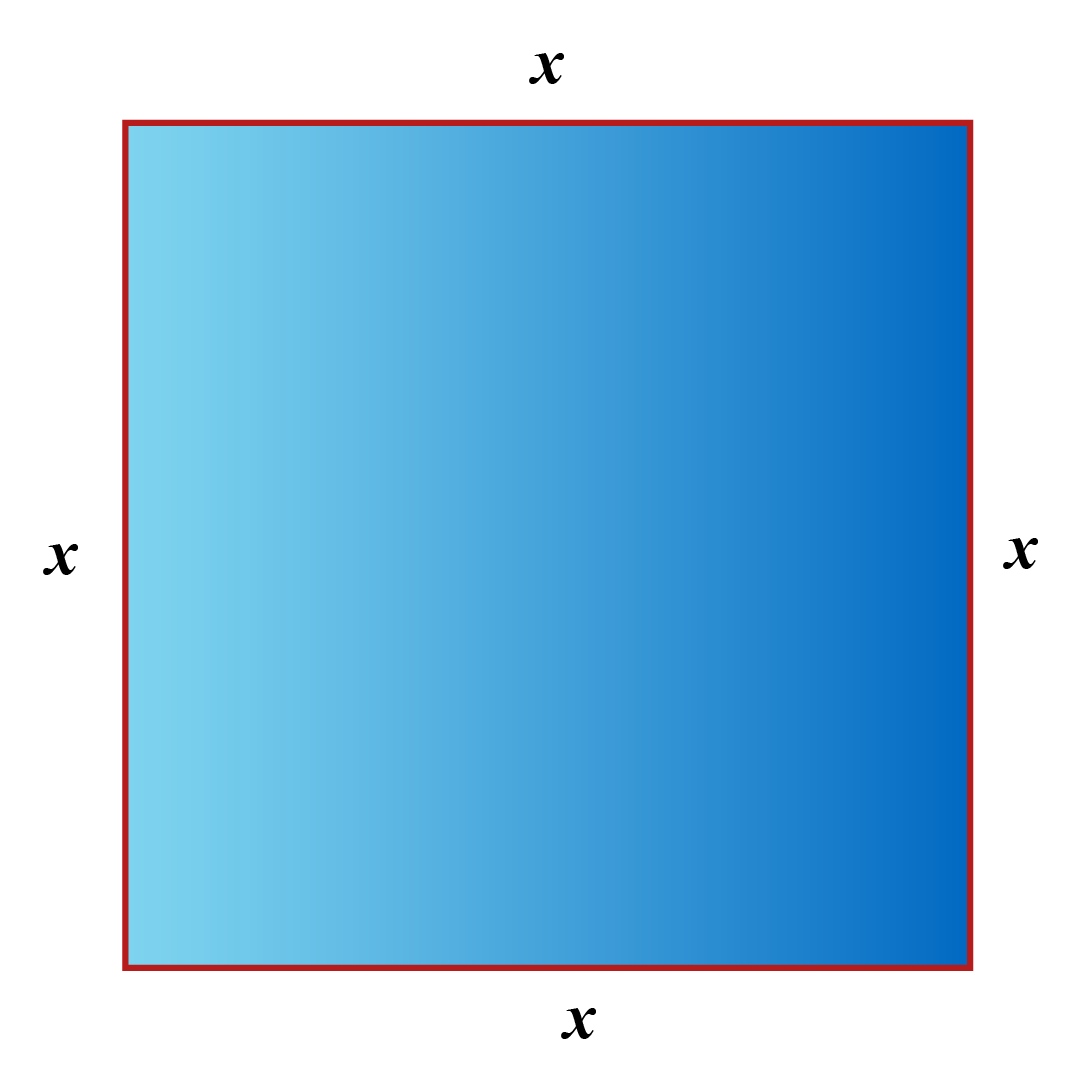$$x^2$$
Rectangle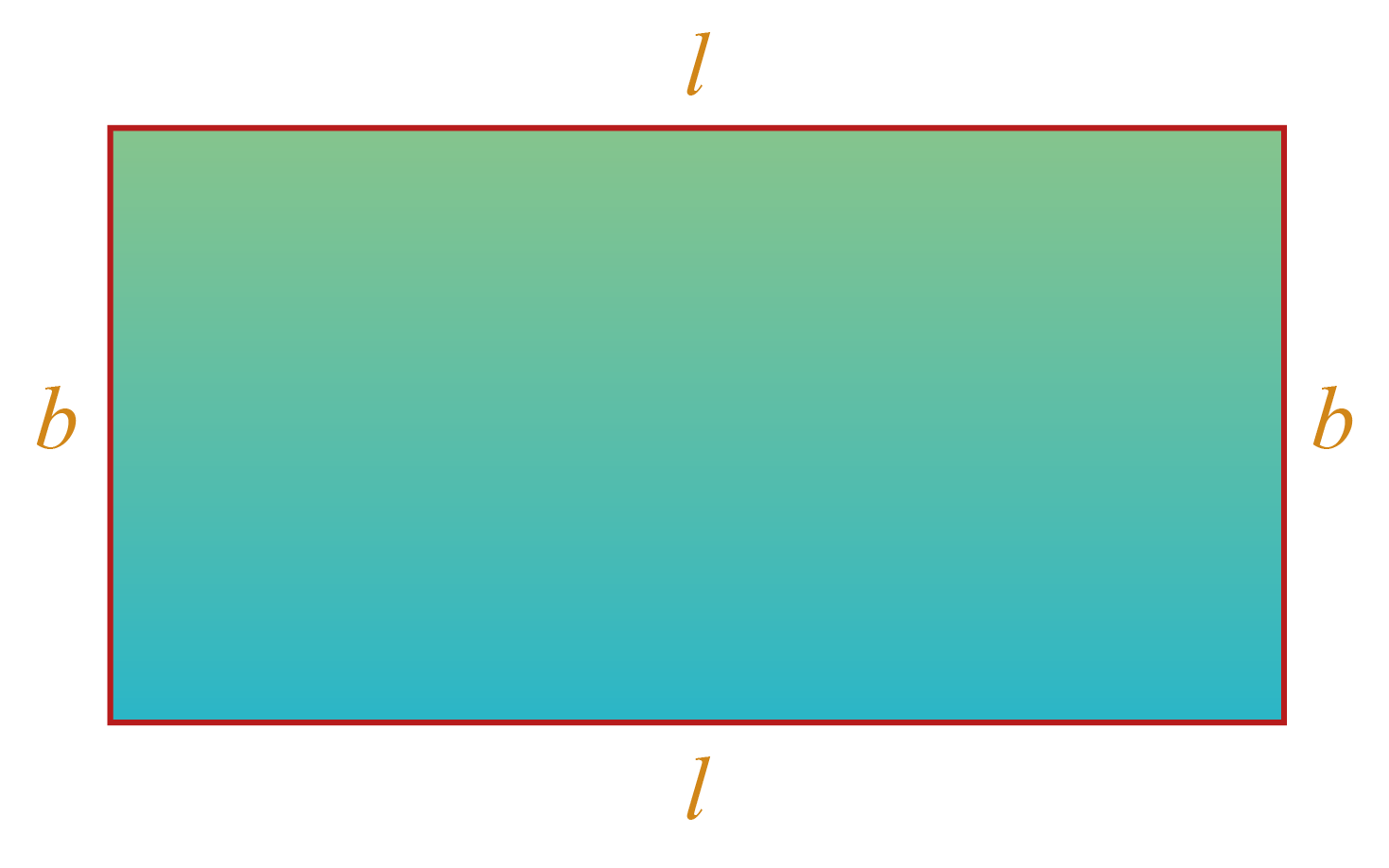$$l \times b$$

Circle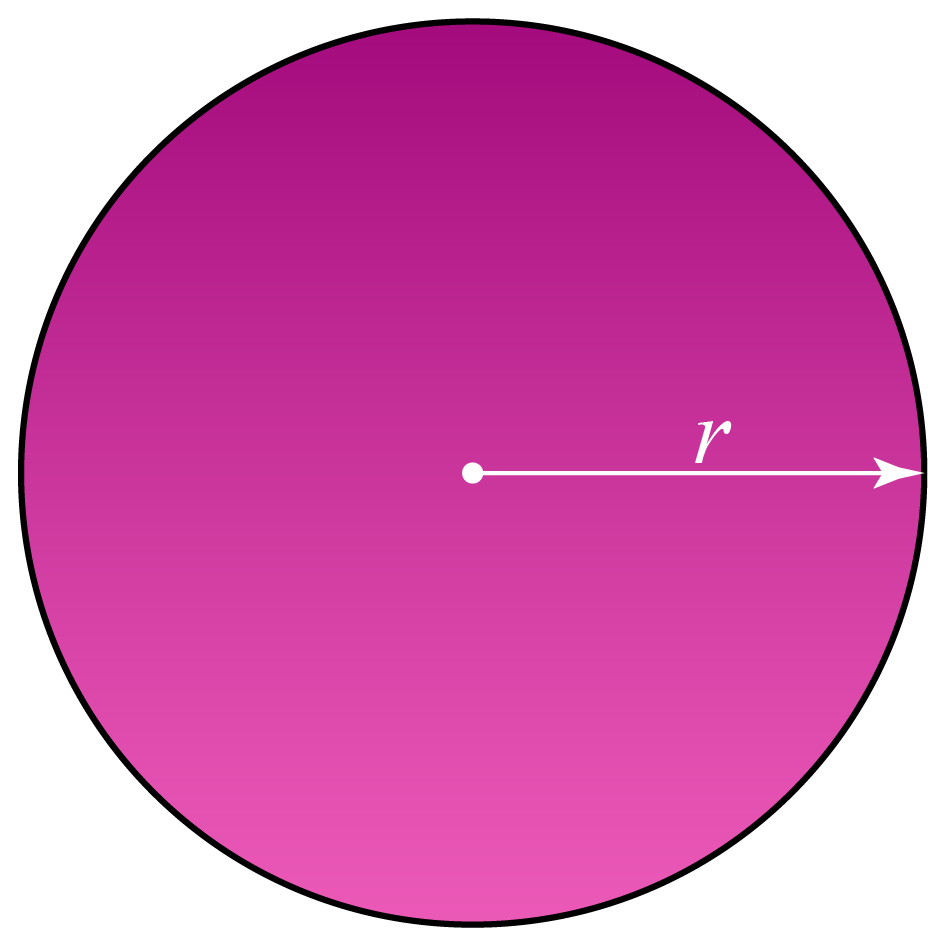$$\pi \text{r}^2$$

Triangle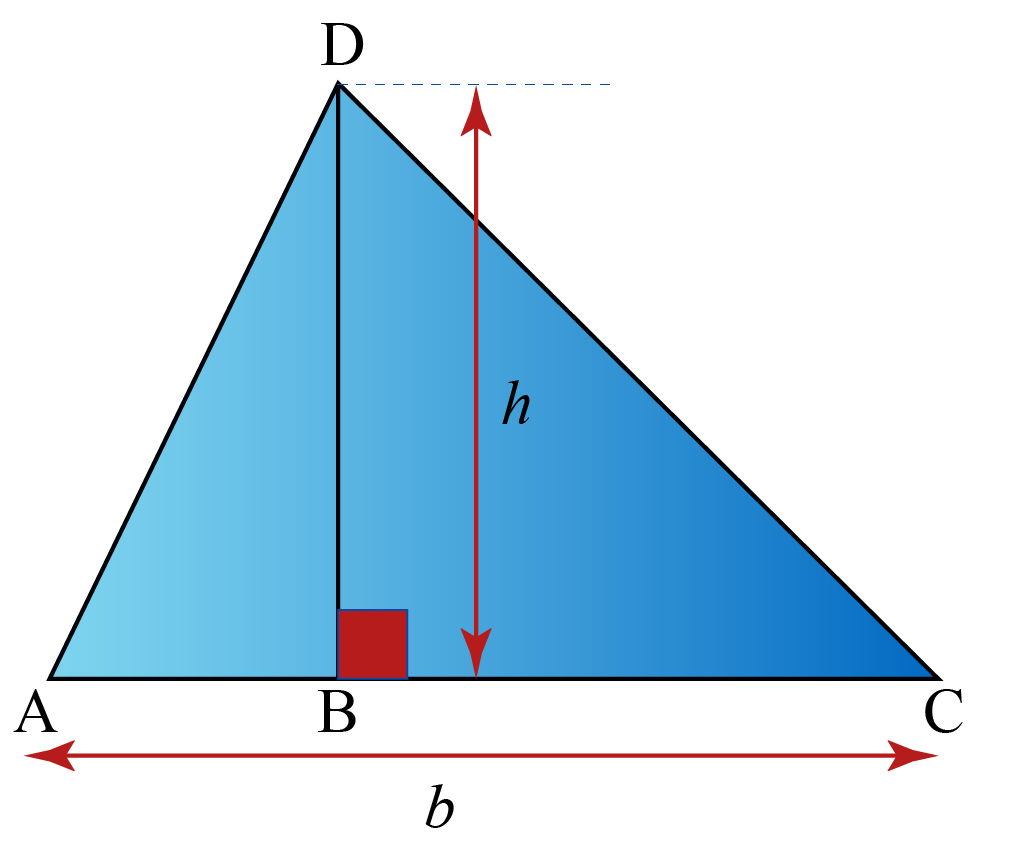$$\frac{1}{2} \times \text{b} \times \text{h}$$

Parallelogram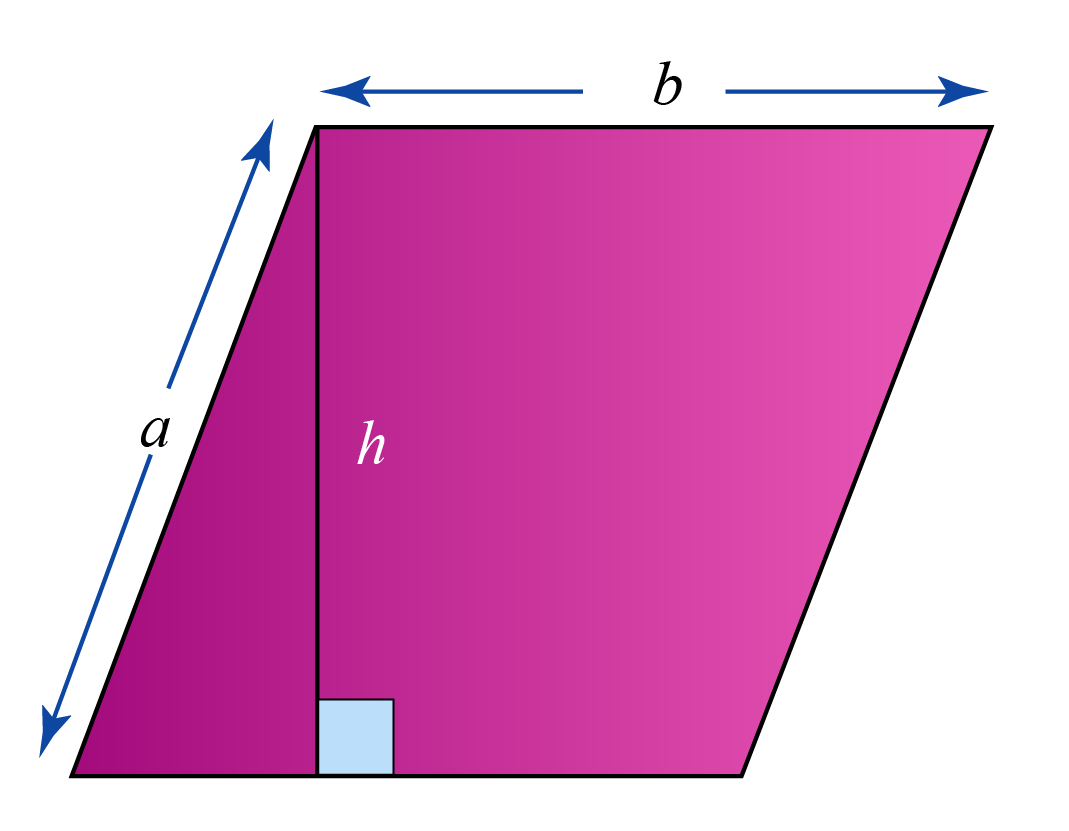$$b \times h$$

Isosceles Trapezoid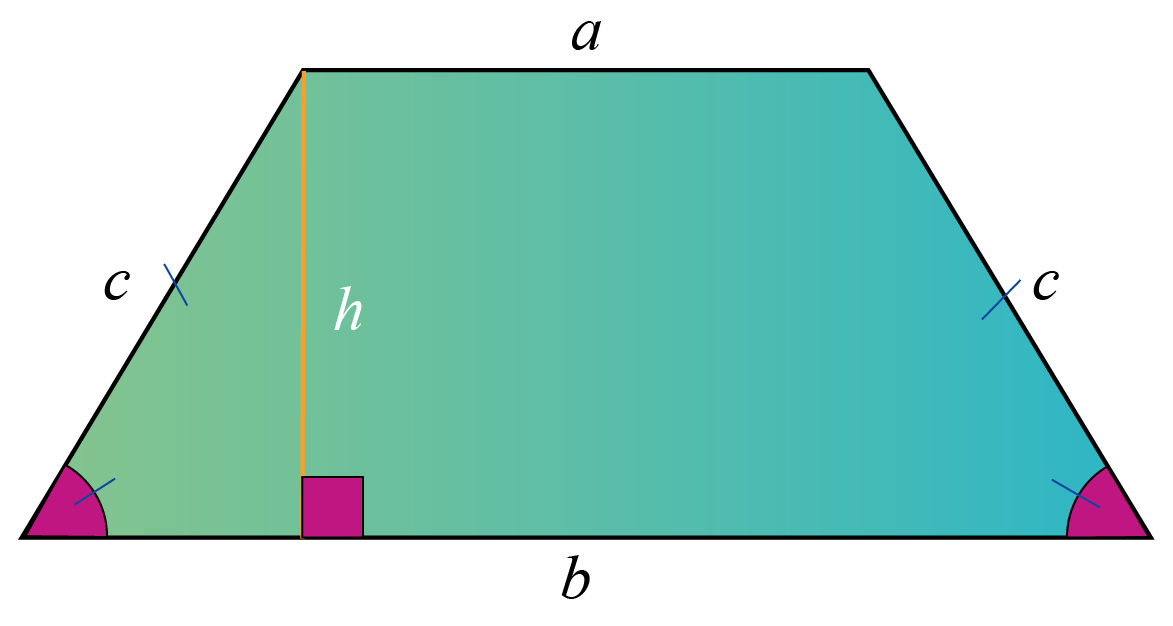$$\frac{1}{2}(a+b)h$$

Rhombus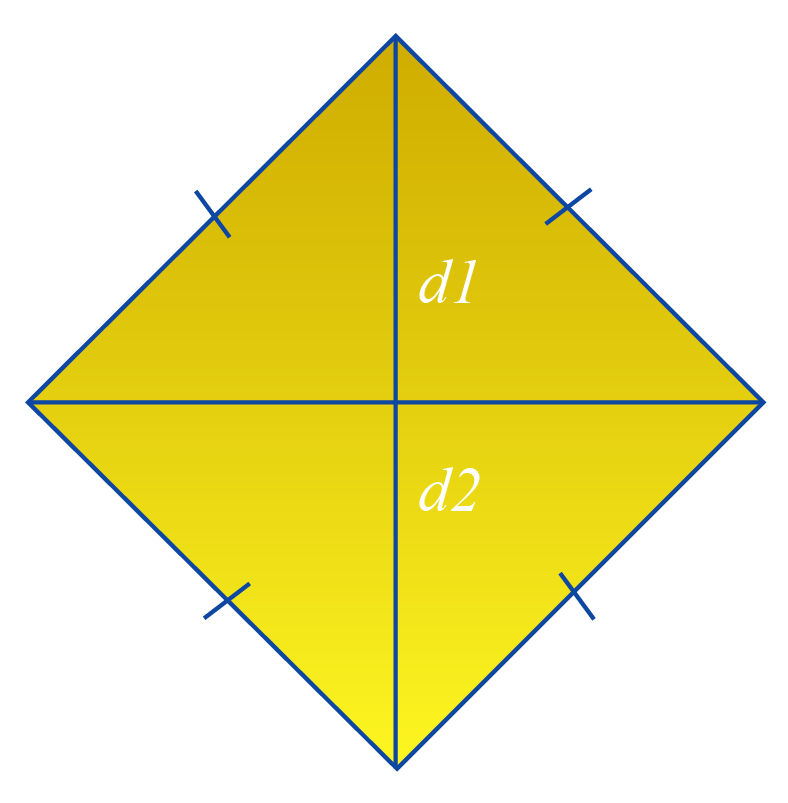$$\frac{1}{2} \times d_1 \times d_2$$

Kite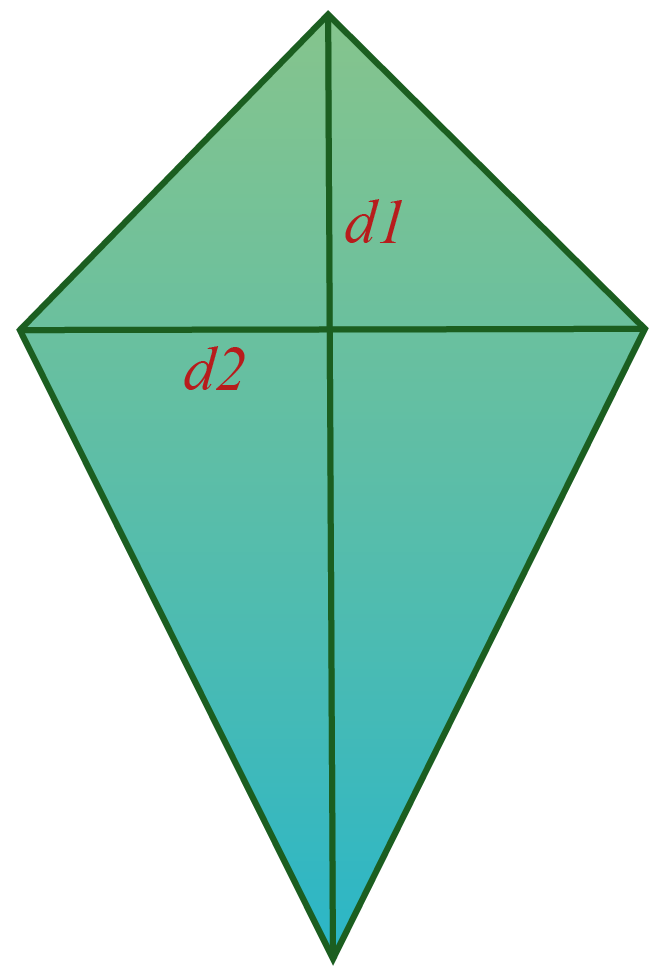$$\frac{1}{2} \times d_1 \times d_2$$

The simulation below shows how the area is calculated in a triangle, square, rectangle and circle.

Try to apply the formula and calculate the area and then verify your answer.

Help your child score higher with Cuemath’s proprietary FREE Diagnostic Test. Get access to detailed reports, customized learning plans, and a FREE counseling session. Attempt the test now.

## Solved Examples

 Example 1

Find the area of a square of side 7 cm.

Solution:

##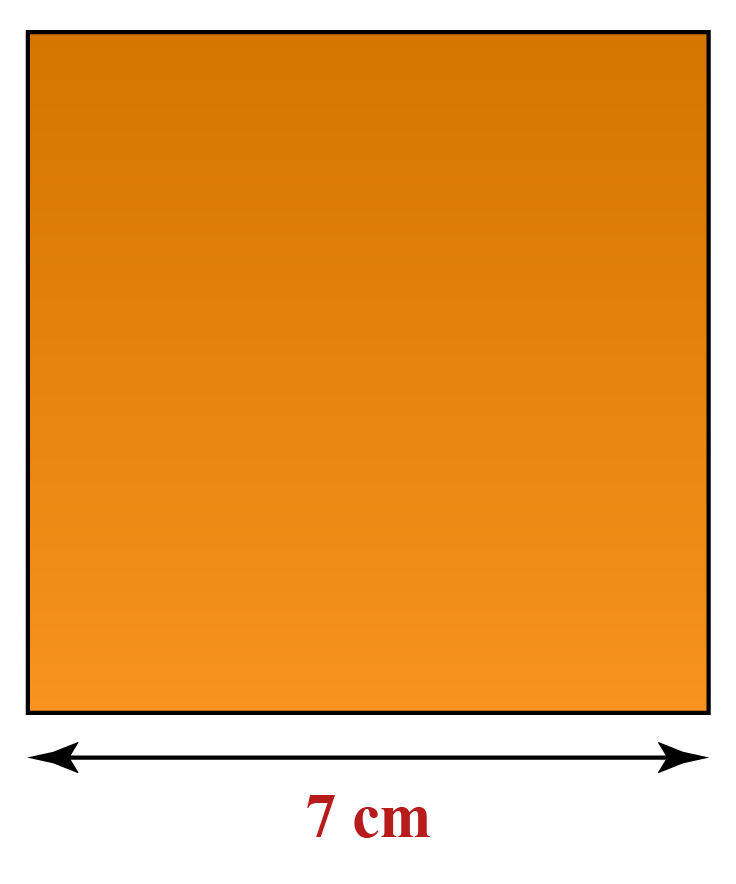Area of a square of side 7 cm is:

\begin{align} 7 \text{ cm} \times 7 \text{ cm}= 49 \;\text{cm}^2 \end{align}

 $$\therefore$$ Area of the square $$= 49 \text { cm}^2$$
 Example 2

The dimensions of a rectangle are 15 cm and 8 cm.

Find its area.

Solution: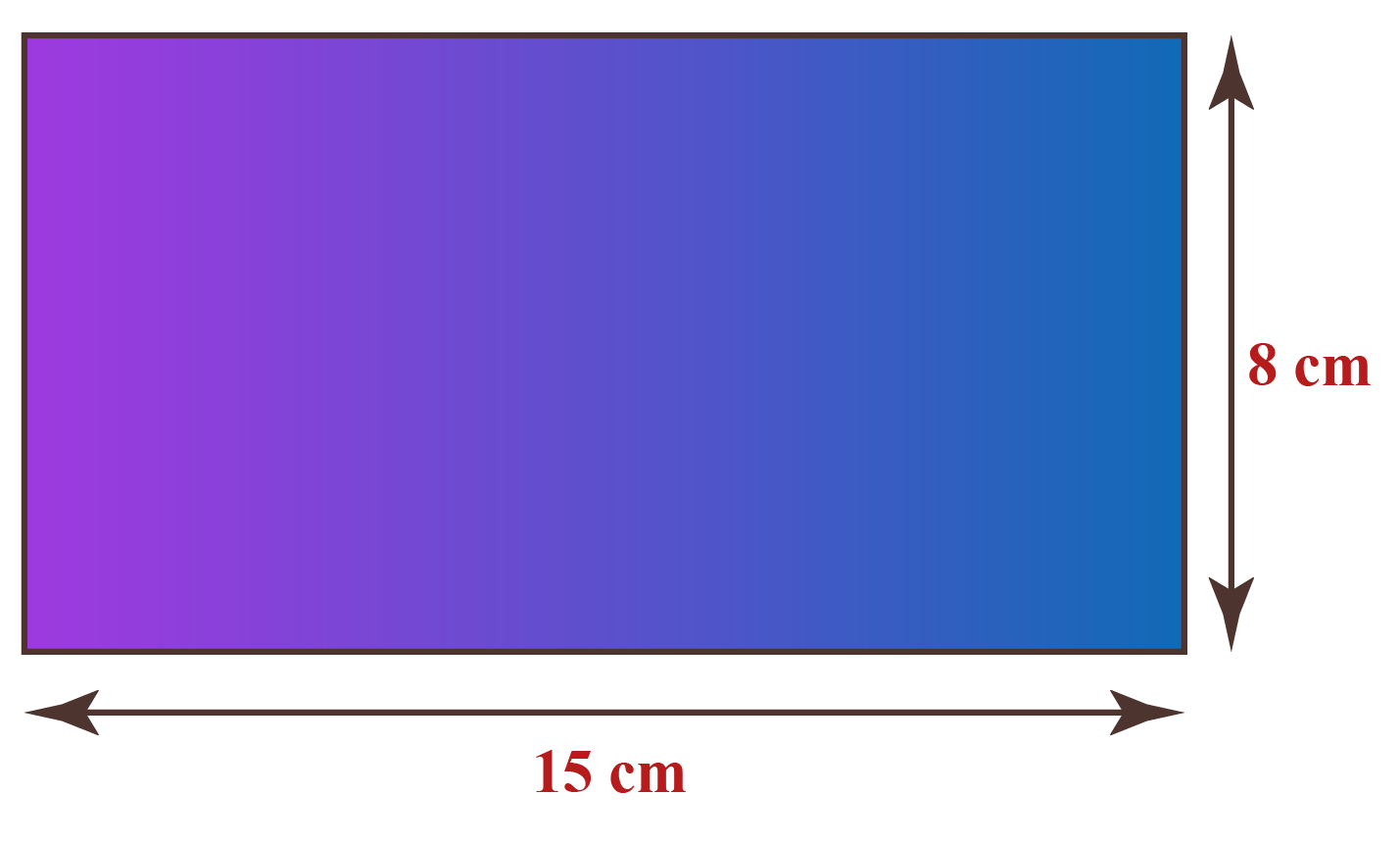Area of a rectangle is the product of its length and breadth.

Area = $$15 \times 8= 120\text{ cm}^2$$

 $$\therefore$$ The area of the rectangle is $$= 120 \text { cm}^2$$
 Example 3

Find the area of a circle with radius 14 cm.

Solution:

##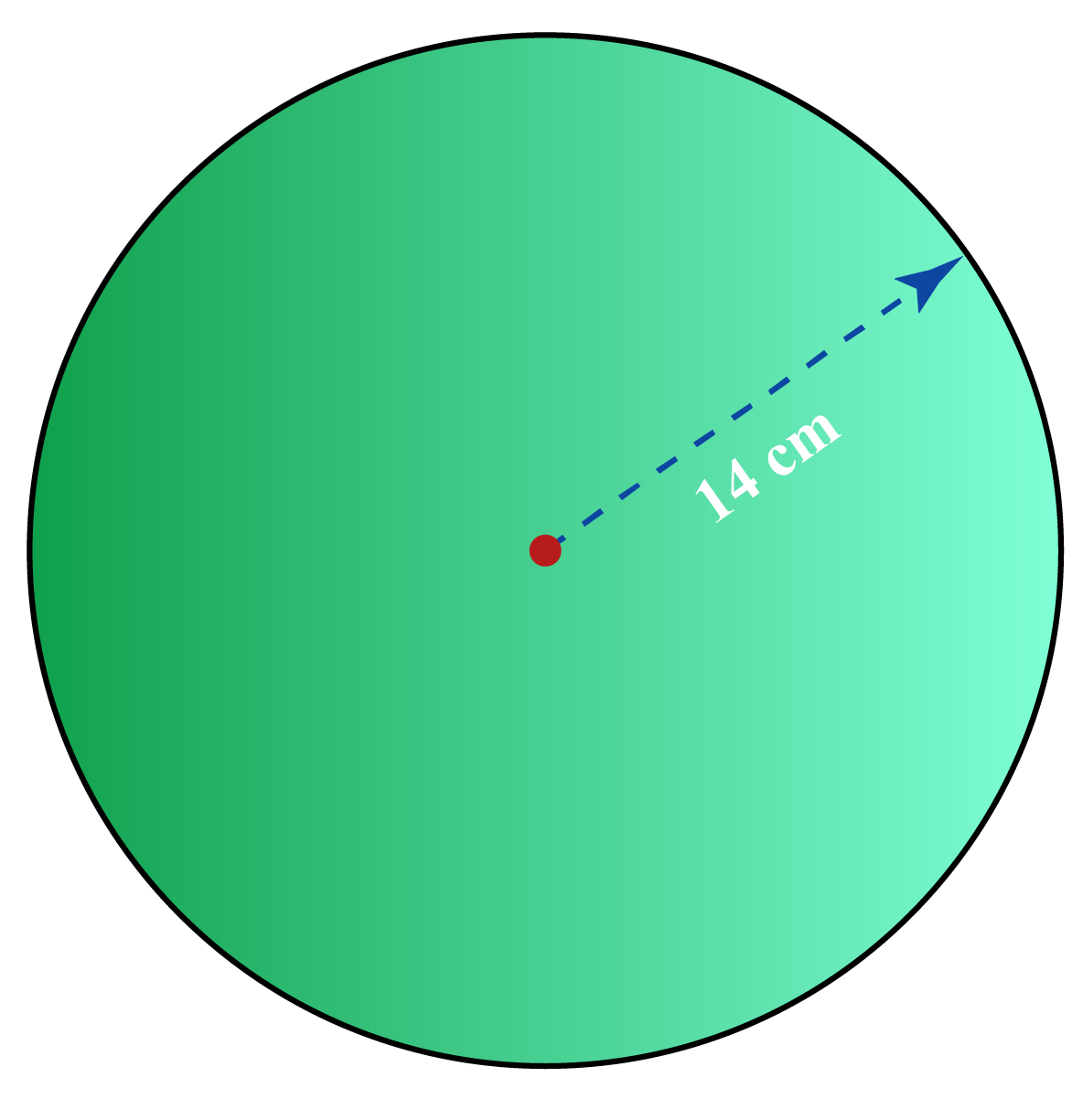Given: Radius of the circle = 14 cm

Area of a circle is calculated by the following formula

\begin{align} &= \pi r^2 \\ &= \left(\frac{22}{7}\right) \times \left({14}\right) \times \left({14}\right) \\ &= \text{616} \text{ cm}^2 \end{align}

 $$\therefore$$ Area of the circle $$= 616 \text { cm}^2$$
 Example 4

Calculate the area of the given shape by counting the squares.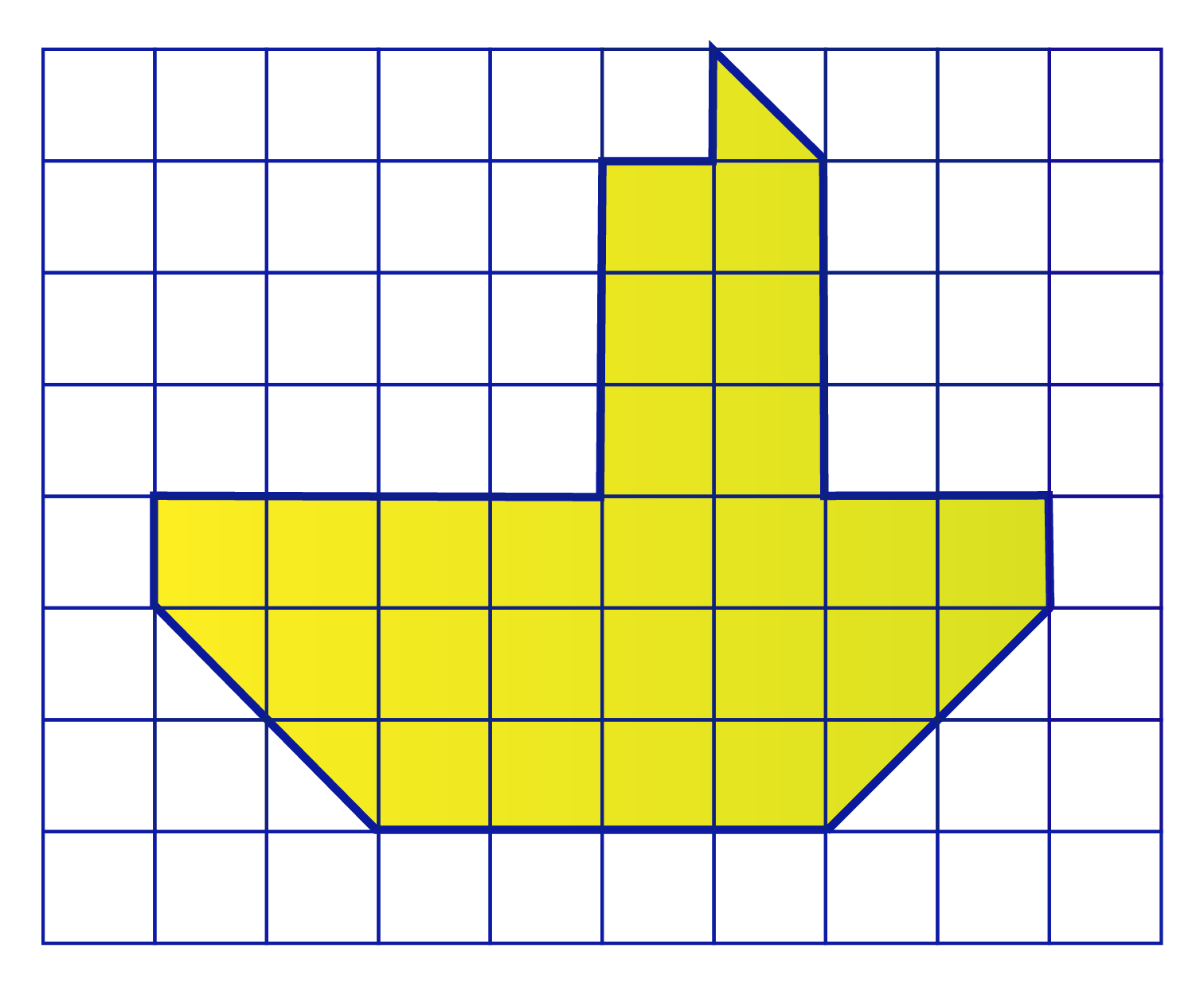Solution:

Let's count all the full squares and half squares.

We can combine two half squares into 1 sq. unit.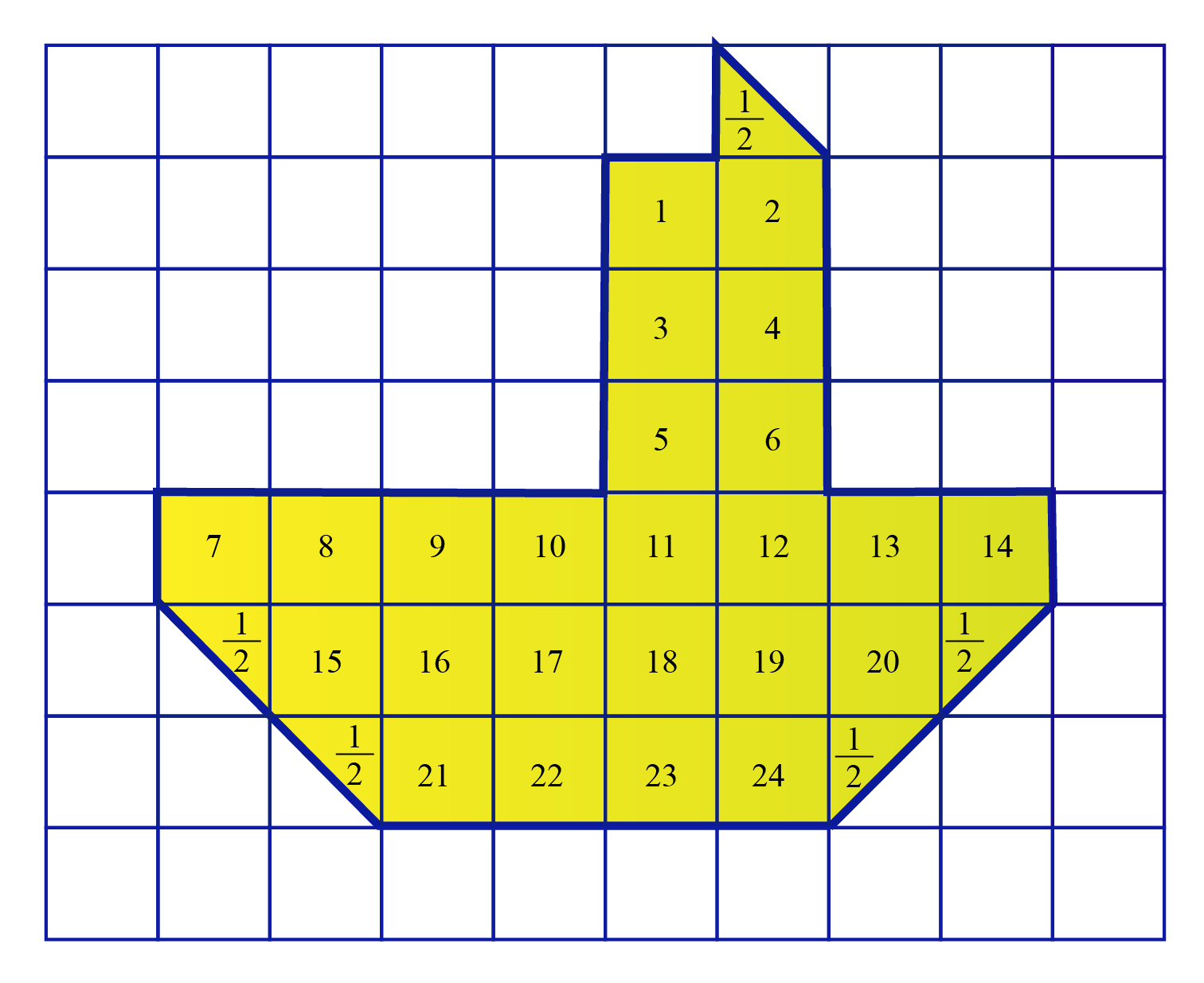There are 24 unit squares and 5 half squares which add up to $$26\dfrac{1}{2}$$ sq. units.

 $$\therefore$$ Area of the given shape $$= 26\dfrac{1}{2} \text {sq. units}$$

## Area - Measuring Units

Area is calculated in terms of the shape's length and breadth.

Length is unidimensional and measured in units such as centimetre (cm), metre (m), kilometre (km), feet (ft), yards (yd), inches (in), etc.

However, the area of a shape is a two-dimensional quantity.

Hence, it is measured in square units like sq. cm or (cm2), sq. in or (in2), sq. ft or (ft 2), sq. m or (m2), etc.Challenging Questions
1. Here is an activity for you.
A rectangle of side 5 X 6 units is given.
Can you make a compound shape such that its area is 15 units2

Have a doubt that you want to clear? Get it clarified with simple solutions on Area of a Shape from our Math Experts at Cuemath’s LIVE, Personalised and Interactive Online Classes.

Make your kid a Math Expert, Book a FREE trial class today!

## Practice Questions

Here are a few activities for you to practice. Select/Type your answer and click the "Check Answer" button to see the result.Tips and Tricks
1. We often memorise the formulas for calculating the area of shapes.
An easier method would be to use grid lines to understand how the formula has been derived.
2. We often get confused between area and perimeter of a shape.
A thorough understanding can be built by tracing the surface of any shape and observing that the area is essentially the space or the region covered by the shape.

IMO (International Maths Olympiad) is a competitive exam in Mathematics conducted annually for school students. It encourages children to develop their math solving skills from a competition perspective.

## 1. What is the formula for finding the area?

• The formula for finding the area of a square is $$s^2$$ where s is the length of the side.
• The area of a rectangle is the product of its length ($$l$$) and breadth($$b$$) $$\text{Area} = l \times b$$
• The area of a triangle is half times the product of its base ($$b$$) and its height ($$h$$); $$\text{Area} = \frac{1}{2} \times h \times b$$
• The area of a circle is given by the formula $$\pi r^2$$ where $$r$$ is the radius of the circle and $$\pi$$ is the constant 3.14.

## 2. What is the formula for area of all shapes?

The formula for the commonly used shapes is listed in the section Area of Shapes -Formula.

The simulation also explains how to apply the formula to calculate the area of different shapes.

## 3. How do you find the area in math problems?

To find the area in math problems, we can either count the number of unit squares occupied by the shape in the grid or apply the formula listed in the table under the section Area of Shapes -Formula.

More Important Topics
Numbers
Algebra
Geometry
Measurement
Money
Data
Trigonometry
Calculus